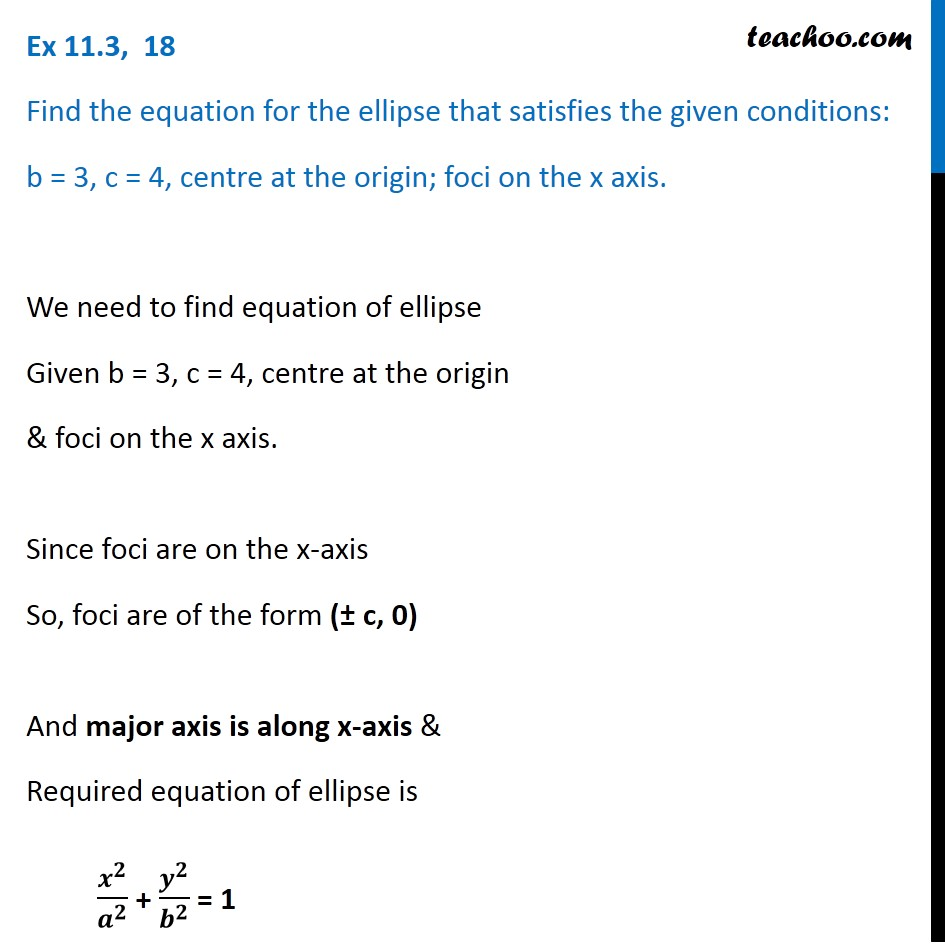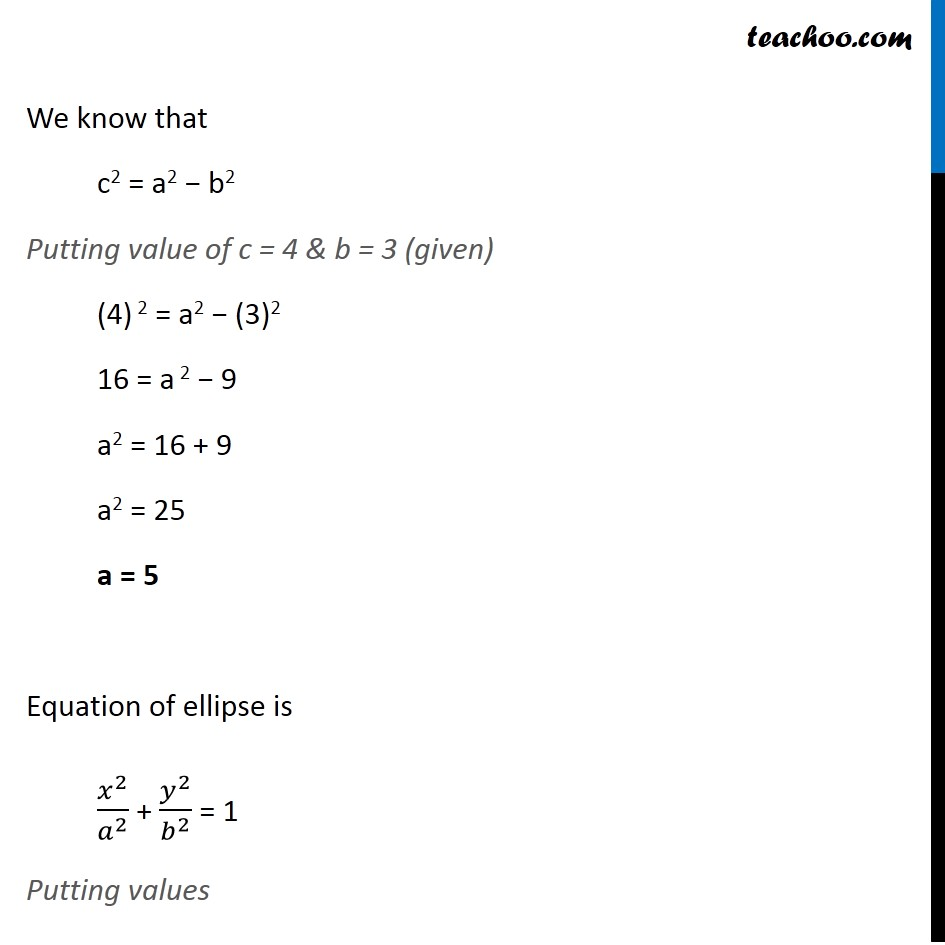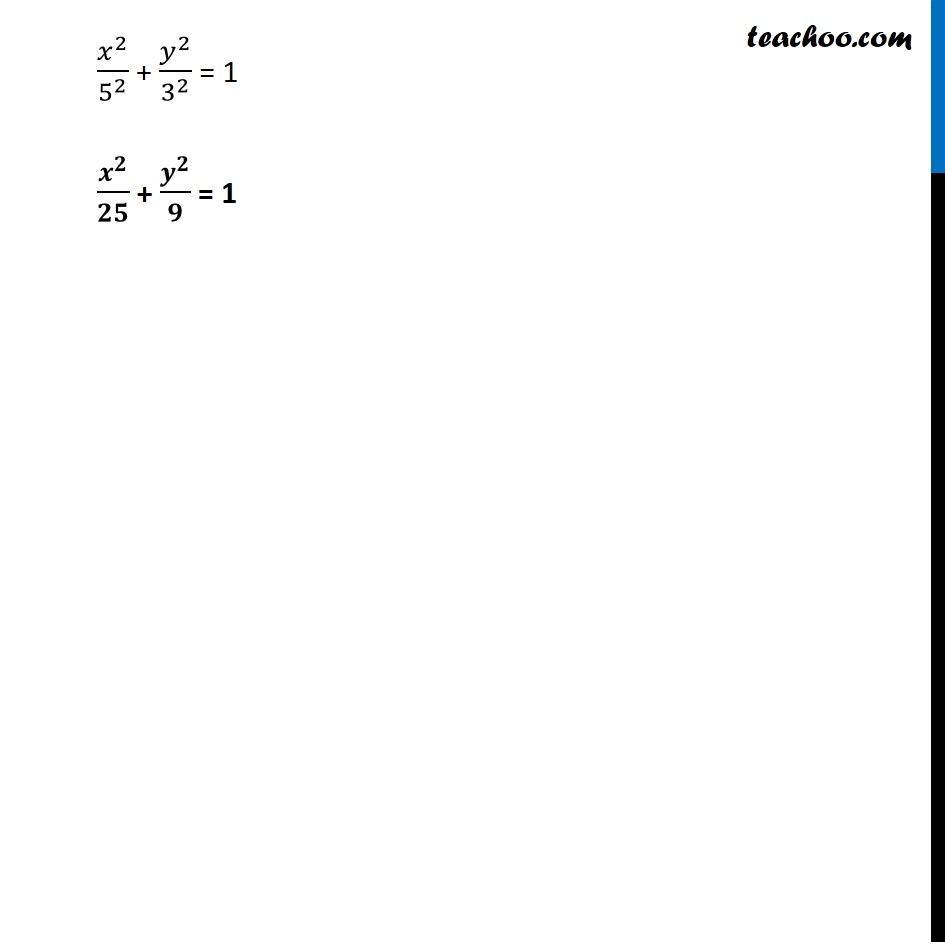Ex 10.3

Chapter 10 Class 11 Conic Sections
Serial order wiseLearn in your speed, with individual attention - Teachoo Maths 1-on-1 Class

### Transcript

Ex 10.3, 18 Find the equation for the ellipse that satisfies the given conditions: b = 3, c = 4, centre at the origin; foci on the x axis. We need to find equation of ellipse Given b = 3, c = 4, centre at the origin & foci on the x axis. Since foci are on the x-axis So, foci are of the form (± c, 0) And major axis is along x-axis & Required equation of ellipse is 𝒙^𝟐/𝒂^𝟐 + 𝒚^𝟐/𝒃^𝟐 = 1 We know that c2 = a2 − b2 Putting value of c = 4 & b = 3 (given) (4) 2 = a2 − (3)2 16 = a 2 − 9 a2 = 16 + 9 a2 = 25 a = 5 Equation of ellipse is 𝑥^2/𝑎^2 + 𝑦^2/𝑏^2 = 1 Putting values 𝑥^2/5^2 + 𝑦^2/3^2 = 1 𝒙^𝟐/𝟐𝟓 + 〖𝒂𝒚〗^𝟐/𝟗 = 1